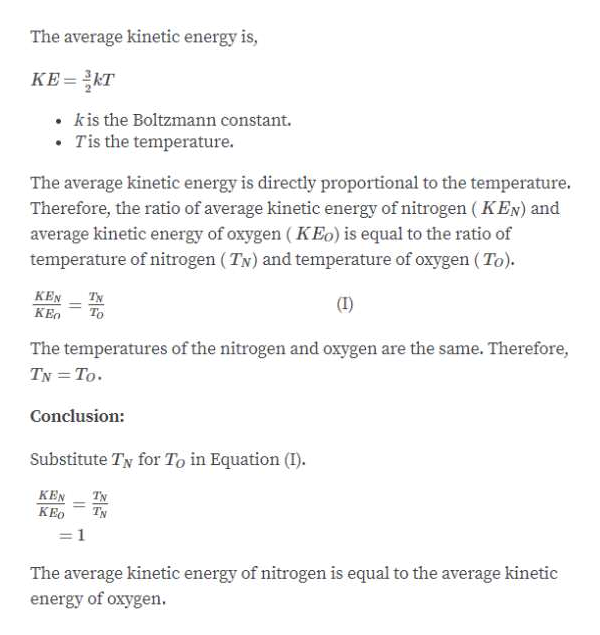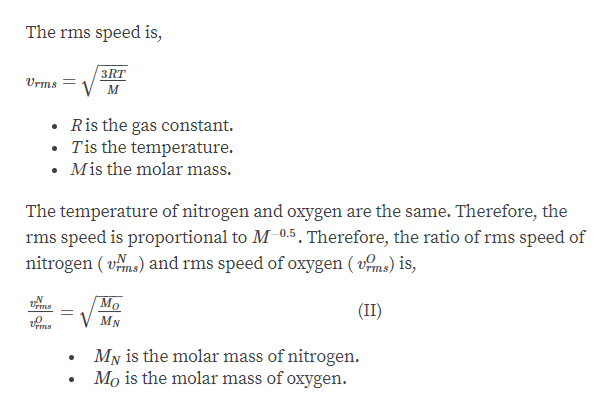# The air we breathe is largely composed of nitrogen (N2) and oxygen (O2) molecules. The mass of an N2 molecule is less than the mass of an O2 molecule. (a) For air at 300 K, is the average kinetic energy of an N2 molecule greater than, less than, or equal to the average kinetic energy of an O2 molecule? (b) Is the rms speed in air of an N2 molecule greater than, less than, or equal to the rms speed in air of an O2 molecule?

Question
7 views

The air we breathe is largely composed of nitrogen (N2) and oxygen (O2) molecules. The mass of an N2 molecule is less than the mass of an O2 molecule. (a) For air at 300 K, is the average kinetic energy of an N2 molecule greater than, less than, or equal to the average kinetic energy of an O2 molecule? (b) Is the rms speed in air of an N2 molecule greater than, less than, or equal to the rms speed in air of an O2 molecule?

check_circle

Step 1

Part A:help_outlineImage TranscriptioncloseThe average kinetic energy is, KE = KT • kis the Boltzmann constant. • Tis the temperature. The average kinetic energy is directly proportional to the temperature. Therefore, the ratio of average kinetic energy of nitrogen ( KEN) and average kinetic energy of oxygen ( KEo) is equal to the ratio of temperature of nitrogen (TN) and temperature of oxygen ( To). KEN KEO TN To (I) The temperatures of the nitrogen and oxygen are the same. Therefore, TN = To. Conclusion: Substitute Ty for To in Equation (I). KEN KEO TN TN :1 The average kinetic energy of nitrogen is equal to the average kinetic energy of oxygen. fullscreen
Step 2

Part B :...help_outlineImage TranscriptioncloseThe rms speed is, 3RT Urms = Ris the gas constant. Tis the temperature. Mis the molar mass. The temperature of nitrogen and oxygen are the same. Therefore, the rms speed is proportional to M0.5 , Therefore, the ratio of rms speed of nitrogen ( vms) and rms speed of oxygen ( vms) is, (II) My is the molar mass of nitrogen. Mo is the molar mass of oxygen. fullscreen

### Want to see the full answer?

See Solution

#### Want to see this answer and more?

Solutions are written by subject experts who are available 24/7. Questions are typically answered within 1 hour.*

See Solution
*Response times may vary by subject and question.
Tagged in

### Other